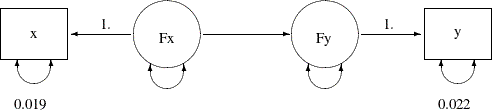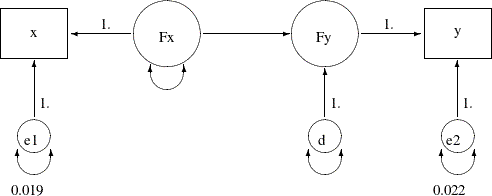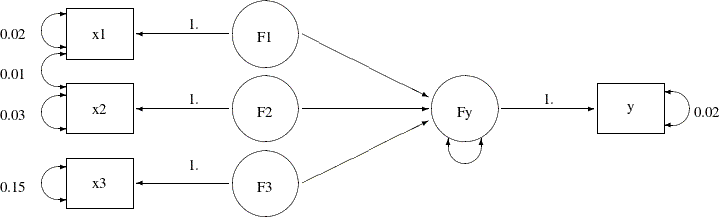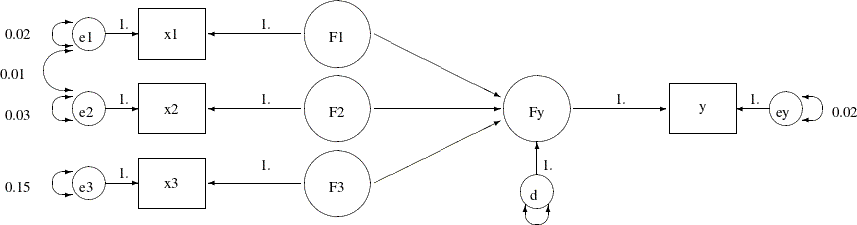### Example 27.11 Measurement Error Models Specified As Linear Equations

In Example 27.8, you fit a simple measurement error model with errors in both of the predictor variable `x` and the outcome variable `y`. From prior studies, the measurement error variance of `x` is 0.019 and the measurement error variance of `y` is 0.022. You use the following path diagram to represent the model:

Output 27.11.1:With this path diagram, you use the PATH modeling language of PROC CALIS to specify the model, as shown in the following:

```proc calis data=measures;
path
x  <=== Fx  = 1.,
Fx ===> Fy   ,
Fy ===> y   = 1.;
pvar
x  = 0.019,
y  = 0.022,
Fx Fy;
run;
```

In the path diagram and in the PATH model specification, there are no explicit representations of the error terms in the model. You express the error variances of `x`, `y`, and `Fy` as partial variances of the endogenous variables. In the path diagram, you represent these partial variances by the double-headed arrows. Correspondingly, in the PATH statement of PROC CALIS, you specify these partial variances in the PVAR statement.

In practice, some researchers might prefer to express the error terms in the model explicitly. For example, with the error terms added to the preceding measurement error model, the new path diagram becomes:

Output 27.11.2:In the path diagram, you add paths from error variables `e1`, `e2`, and `d` to the endogenous variables `x`, `y`, and `Fy`, respectively. All these paths from the error terms have a fixed path coefficient of 1. The error variances are represented by double-headed arrows directly attached to them. For example, the variance of `e1` is fixed at 0.019, and the variance of `e2` is fixed at 0.022. The variance of `d`, which is sometime called the disturbance, is a free unnamed parameter in the path diagram. Similarly, the variance of `Fx` is a free unnamed parameter in the model.

Corresponding to this new path diagram, you can use the LINEQS modeling language for specifying your model in PROC CALIS, as shown in the following statements:

```proc calis data=measures;
lineqs
x   = 1. * Fx  +  e1,
y   = 1. * Fy  +  e2,
Fy  =    * Fx  +   d;
variance
e1  = 0.019,
e2  = 0.022,
Fx d;
run;
```

The LINEQS model specification in PROC CALIS emphasizes the linear equation input. In each of the linear equations in the LINEQS statement, you specify an endogenous variable and how it is related to other variables. An endogenous variable in the path diagram is a variable that has at least one single-headed arrow pointing to it. You need to list all endogenous variables on the left-hand side of the linear equations of the LINEQS statement. In the current model, variables `x`, `y`, and `Fy` are endogenous, and therefore you specify three linear equations in the LINEQS statement. The first two equations represent the measurement model for the observed variables, while the third equation represents the structural equation of the model. Notice that in the third equation, you do not specify the path coefficient that is attached to `Fx`. PROC CALIS treats unspecified path coefficients as free parameters. The effect of `Fx` on `Fy` is freely estimated, as required in the path diagram representation.

In the VARIANCE statement, you specify the variances of the exogenous variables in the model. The specifications in the VARIANCE statement of the LINEQS model are very similar to those in the PVAR statement of the PATH model. The main difference is the use of error variable names in the VARIANCE statement. With the LINEQS model specification, you can only specify exogenous variables in the VARIANCE statement. Hence, you must specify the error variables `e1`, `e2`, and `d` in the VARIANCE statement of the LINEQS model, instead of the corresponding endogenous variables `x`, `y`, and `Fy` in the PVAR statement of the PATH model.

Output 27.11.3 shows the parameter estimates of the LINEQS model.

Output 27.11.3: LINEQS Parameter Estimates of the Measurement Model for the Measures Data

Linear Equations
x =   1.0000   Fx + 1.0000   e1
y =   1.0000   Fy + 1.0000   e2
Fy =   1.1791 * Fx + 1.0000   d
Std Err     0.1029   _Parm1
t Value     11.4615

Estimates for Variances of Exogenous Variables
Variable
Type
Variable Parameter Estimate Standard
Error
t Value
Error e1   0.01900
e2   0.02200
Latent Fx _Parm2 0.78062 0.20999 3.71741
Disturbance d _Parm3 0.18486 0.06126 3.01755

All these estimates are essentially the same as those obtained from the PATH model specification, as shown in Output 27.11.4.

Output 27.11.4: PATH Parameter Estimates of the Measurement Model for the Measures Data

PATH List
Path Parameter Estimate Standard
Error
t Value
x <=== Fx   1.00000
Fx ===> Fy _Parm1 1.17914 0.10288 11.46153
Fy ===> y   1.00000

Variance Parameters
Variance
Type
Variable Parameter Estimate Standard
Error
t Value
Error x   0.01900
y   0.02200
Exogenous Fx _Parm2 0.78062 0.20999 3.71741
Error Fy _Parm3 0.18486 0.06126 3.01755

You can use either the LINEQS or PATH model specification in PROC CALIS for your analysis problems. They give you the same estimation results.

So far the measurement error model is concerned with one predictor. With more predictors in the model, you might also want to model the correlated measurement errors in the `x` variables. You can analyze this kind of model by using the PATH model specification, as shown in Example 27.10. With measurement error terms explicitly assumed, you can also use the LINEQS model specification. This example illustrates how you can do that by using the same data set and the measurement error model with correlated errors in Example 27.10.

In the data set, you have four observed variables: `x1``x3` and `y`. All are measured with errors, as represented by the following path diagram:

Output 27.11.5:In the path diagram, `F1``F3` and `Fy` represent true scores for the measurement indicators `x1``x3` and `y`, respectively. You predict `Fy` by `F1``F3`, which represents the structural relationships in the model. Measurement error variances of the observed variables are treated as known and are represented by the double-headed arrows attached to the observed variables. For example, the error variance of `x3` is 0.15. In addition, the error covariance between `x1` and `x2` is treated as known. The double-headed arrow that connects `x1` and `x2` represents the error covariance, and this covariance is fixed at 0.01 in the model.

You transcribe this path diagram representation into the following PATH model specification:

```proc calis data=multiple nobs=37;
path
Fy  <===   F1 F2 F3,
F1  ===>   x1   = 1.,
F2  ===>   x2   = 1.,
F3  ===>   x3   = 1.,
Fy  ===>   y    = 1.;
pvar
x1 x2 x3 y = .02 .03 .15 .02,
Fy;
pcov
x1 x2 = 0.01;
run;
```

To represent the error terms explicitly, you can add the error terms to the path diagram with some modifications, as shown in the following:

Output 27.11.6:In the path diagram, you attach error variables `e1``e3`, `ey`, and `d` to the associated endogenous variables in the model. The error variances and covariances, which are attached to the endogenous variables directly, are now attached to the corresponding error variables. With this new path diagram, you can use the following LINEQS model specification for the model:

```proc calis data=multiple nobs=37;
lineqs
Fy =     * F1 +  * F2 +  * F3 + d,
x1 =  1. * F1 + e1,
x2 =  1. * F2 + e2,
x3 =  1. * F3 + e3,
y =  1. * Fy + ey;
variance
e1-e3 ey = .02 .03 .15 .02,
d;
cov
e1 e2 = 0.01;
run;
```

Again, in each linear equation of the LINEQS statement, you specify the functional relationship of an endogenous variable with other variables, including the error variable. The first equation is the structural equation in the model. You want to estimate the effects of `F1`, `F2`, and `F3` on `Fy`. The error or disturbance variable is `d`. In the next four equations, you relate the observed variables with their true scores counterparts.

In the VARIANCE statement, you specify the error variances with reference to the error variables in the path diagram. Four of the error variances are fixed constants, as required in the model. The last specification represents a free parameter for the variance of `d`. The specifications in the VARIANCE statement of the LINEQS model are similar to those in the PVAR statement of the PATH model specification. The difference is that in the PATH model specification the reference variables are the endogenous variables in the PATH model, while in the LINEQS model specification the reference variables are the associated error variables.

In the COV statement, you specify the covariance between the error variables `e1` and `e2`. Again, this is similar to the corresponding specification of the PATH model, where the same error covariance is specified as the partial covariance between `x1` and `x2` in the PCOV statement.

Output 27.11.7 shows the parameter estimates that result from using the LINEQS model specification. Estimates in the equations, variances, and covariances are shown respectively.

Output 27.11.7: Parameter Estimates of the Measurement Model with Multiple Predictors: LINEQS Model

Linear Equations
Fy =   0.4684 * F1 + 0.0455 * F2 + 0.1369 * F3 + 1.0000   d
Std Err     0.2269   _Parm1   0.0707   _Parm2   0.0719   _Parm3
t Value     2.0639       0.6431       1.9035
x1 =   1.0000   F1 + 1.0000   e1
x2 =   1.0000   F2 + 1.0000   e2
x3 =   1.0000   F3 + 1.0000   e3
y =   1.0000   Fy + 1.0000   ey

Estimates for Variances of Exogenous Variables
Variable
Type
Variable Parameter Estimate Standard
Error
t Value
Error e1   0.02000
e2   0.03000
e3   0.15000
ey   0.02000
Disturbance d _Parm4 0.16421 0.04523 3.63046
Latent F1 _Add1 1.40000 0.33470 4.18289

Covariances Among Exogenous Variables
Var1 Var2 Parameter Estimate Standard
Error
t Value
e1 e2   0.01000
F2 F1 _Add4 0.17000 0.21508 0.79039
F3 F1 _Add5 4.21000 1.02416 4.11070
F3 F2 _Add6 0.58000 0.67829 0.85509

Output 27.11.8 shows the parameter estimates that result from using the PATH model specification. The estimates in the path list shown in Output 27.11.8 correspond to those of the equation output in Output 27.11.7. The variance estimates in Output 27.11.8 correspond to those variance estimates of the exogenous variables of the LINEQS model, as shown in Output 27.11.7. Finally, the last two tables in Output 27.11.8 correspond to the covariance estimates among the exogenous variables of the LINEQS model, as shown in Output 27.11.7. Again, the LINEQS and PATH model specification give you exactly the same estimation results, but in different output formats.

Output 27.11.8: Parameter Estimates of the Measurement Model with Multiple Predictors: PATH Model

PATH List
Path Parameter Estimate Standard
Error
t Value
Fy <=== F1 _Parm1 0.46839 0.22695 2.06386
Fy <=== F2 _Parm2 0.04549 0.07074 0.64306
Fy <=== F3 _Parm3 0.13694 0.07194 1.90351
F1 ===> x1   1.00000
F2 ===> x2   1.00000
F3 ===> x3   1.00000
Fy ===> y   1.00000

Variance Parameters
Variance
Type
Variable Parameter Estimate Standard
Error
t Value
Error x1   0.02000
x2   0.03000
x3   0.15000
y   0.02000
Fy _Parm4 0.16421 0.04523 3.63046
Exogenous F1 _Add1 1.40000 0.33470 4.18289

Covariances Among Exogenous Variables
Var1 Var2 Parameter Estimate Standard
Error
t Value
F2 F1 _Add4 0.17000 0.21508 0.79039
F3 F1 _Add5 4.21000 1.02416 4.11070
F3 F2 _Add6 0.58000 0.67829 0.85509

Covariances Among Errors
Error of Error of Estimate Standard
Error
t Value
x1 x2 0.01000

In this example, you fit measurement error models by using the LINEQS and PATH model specifications of PROC CALIS. The two different model specification languages give you essentially the same estimation results. The measurement models can have multiple true scores predictors and correlated errors. The measurement error models considered so far have only one measured indicator for each true score latent variable. This is usually not the case in many psychometric or sociological applications where latent factors usually have several observed indicators. The confirmatory factor model is a typical example of this kind of applications. See Example 27.12 for an application of PROC CALIS to fit confirmatory factor models. See Example 27.17 for an application of PROC CALIS to fit a general structural equation model where latent variables have more than one measured indicators.